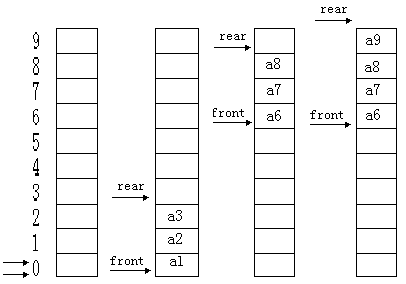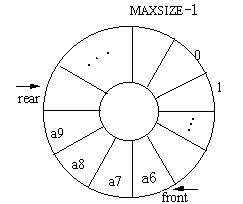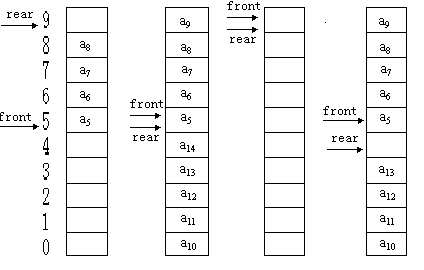# 队列-队列的顺序表示和实现

2014/05/22 20:29## 队列的顺序存储结构

typedef struct {
QElemtype *base;//初始化的动态分配存储空间
int front;//队头指针
int rear;//队尾指针
}SqQueue;

## 循环队列的实现

#include <stdio.h>
#include <stdlib.h>

#define MAXSIZE 10

#define OK 1
#define ERROR 0
#define OVERFLOW -2

typedef int Status;
typedef int QElemtype;

typedef struct {
QElemtype *base;//初始化的动态分配存储空间
int front;//队头指针
int rear;//队尾指针
}SqQueue;//循环队列
//--------------------循环队列的基本操作的算法描述------------------
Status InitQueue(SqQueue *q)
{//构造一个空队列Q
q->base=(QElemtype*)malloc(MAXSIZE*sizeof(QElemtype));
if(!q->base)exit(OVERFLOW);//存储分配失败
q->front= q->rear =0;
return OK;
}

int queuelength(SqQueue q)
{//返回Q的元素个数，即队列的长度
return (q.rear - q.front + MAXSIZE) % MAXSIZE;
}

Status EnQueue (SqQueue *q,QElemtype e)
{//插入元素e为Q的新的队尾元素
if ((q->rear+1) % MAXSIZE == q->front) return ERROR;//队列满，不进行任何操作，不能再入队
q->base[q->rear]=e;
//入队的操作
q->rear=(q->rear+1) % MAXSIZE;
return OK;
}

Status DeQueue (SqQueue *q,QElemtype *e)
{//若队列不空，则删除Q的对头元素，用e返回其值，并返回OK；否则返回ERROR
if (q->front==q->rear) return ERROR;//队列满
*e=q->base[q->front]; //指针的下标运算
q->front=(q->front+1) % MAXSIZE;
return OK;
}

void display_queue(SqQueue *q){
if(q->front==q->rear){
printf("queue is empty!!\n");
}else {
//遍历该循环队列
int front = q->front;
int rear = q->rear;
while(front!=rear){
printf("%d\n",q->base[front]);
++front;
}
}

}

int main(){
SqQueue q;
InitQueue(&q);
int i;
for(i=0;i<11;i++){
if(EnQueue(&q,i)==ERROR){
printf("循环队列已满，该队列长度为9 \n");
}
}
printf("the length of queue is %d \n",queuelength(q)); //the length of queue is 9
int e1,e2,e3,e4,e5;
DeQueue(&q,&e1);
DeQueue(&q,&e2);
DeQueue(&q,&e3);
DeQueue(&q,&e4);
DeQueue(&q,&e5);
printf("%d--%d--%d--%d--%d \n",e1,e2,e3,e4,e5);
printf("the length of queue is %d \n",queuelength(q));
printf("循环队列的遍历\n");
display_queue(&q);
int e6;
DeQueue(&q,&e6);
printf("循环队列的遍历\n");
display_queue(&q);
system("pause");
return 0;
}

循环队列已满，该队列长度为9

the length of queue is 9
0--1--2--3--4
the length of queue is 4

5
6
7
8

6
7
8

x mod y = x % y

========END========

0
2 收藏

### 作者的其它热门文章0 评论
2 收藏
0